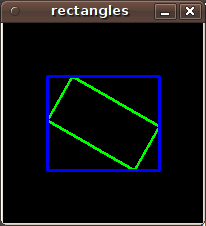OpenCV  4.0.0 Open Source Computer Vision
cv::RotatedRect Class Reference

The class represents rotated (i.e. not up-right) rectangles on a plane. More...

#include "types.hpp"

## Public Member Functions

RotatedRect ()
default constructor More...

RotatedRect (const Point2f &center, const Size2f &size, float angle)

RotatedRect (const Point2f &point1, const Point2f &point2, const Point2f &point3)

Rect boundingRect () const
returns the minimal up-right integer rectangle containing the rotated rectangle More...

Rect_< float > boundingRect2f () const
returns the minimal (exact) floating point rectangle containing the rotated rectangle, not intended for use with images More...

void points (Point2f pts[]) const

## Public Attributes

float angle
returns the rotation angle. When the angle is 0, 90, 180, 270 etc., the rectangle becomes an up-right rectangle. More...

Point2f center
returns the rectangle mass center More...

Size2f size
returns width and height of the rectangle More...

## Detailed Description

The class represents rotated (i.e. not up-right) rectangles on a plane.

Each rectangle is specified by the center point (mass center), length of each side (represented by Size2f structure) and the rotation angle in degrees.

The sample below demonstrates how to use RotatedRect:

Mat test_image(200, 200, CV_8UC3, Scalar(0));
RotatedRect rRect = RotatedRect(Point2f(100,100), Size2f(100,50), 30);
Point2f vertices;
rRect.points(vertices);
for (int i = 0; i < 4; i++)
line(test_image, vertices[i], vertices[(i+1)%4], Scalar(0,255,0), 2);
Rect brect = rRect.boundingRect();
rectangle(test_image, brect, Scalar(255,0,0), 2);
imshow("rectangles", test_image);
waitKey(0);image
CamShift, fitEllipse, minAreaRect, CvBox2D
Examples:
samples/cpp/camshiftdemo.cpp, samples/cpp/fitellipse.cpp, samples/cpp/minarea.cpp, and samples/dnn/text_detection.cpp.

## § RotatedRect() [1/3]

 cv::RotatedRect::RotatedRect ( )

default constructor

## § RotatedRect() [2/3]

 cv::RotatedRect::RotatedRect ( const Point2f & center, const Size2f & size, float angle )

full constructor

Parameters
 center The rectangle mass center. size Width and height of the rectangle. angle The rotation angle in a clockwise direction. When the angle is 0, 90, 180, 270 etc., the rectangle becomes an up-right rectangle.

## § RotatedRect() [3/3]

 cv::RotatedRect::RotatedRect ( const Point2f & point1, const Point2f & point2, const Point2f & point3 )

Any 3 end points of the RotatedRect. They must be given in order (either clockwise or anticlockwise).

## § boundingRect()

 Rect cv::RotatedRect::boundingRect ( ) const

returns the minimal up-right integer rectangle containing the rotated rectangle

## § boundingRect2f()

 Rect_ cv::RotatedRect::boundingRect2f ( ) const

returns the minimal (exact) floating point rectangle containing the rotated rectangle, not intended for use with images

## § points()

 void cv::RotatedRect::points ( Point2f pts[] ) const

returns 4 vertices of the rectangle

Parameters
 pts The points array for storing rectangle vertices. The order is bottomLeft, topLeft, topRight, bottomRight.
Examples:
samples/cpp/fitellipse.cpp, samples/cpp/minarea.cpp, and samples/dnn/text_detection.cpp.

## § angle

 float cv::RotatedRect::angle

returns the rotation angle. When the angle is 0, 90, 180, 270 etc., the rectangle becomes an up-right rectangle.

## § center

 Point2f cv::RotatedRect::center

returns the rectangle mass center

Examples:
samples/cpp/fitellipse.cpp.

## § size

 Size2f cv::RotatedRect::size

returns width and height of the rectangle

Examples:
samples/cpp/fitellipse.cpp.

The documentation for this class was generated from the following file: